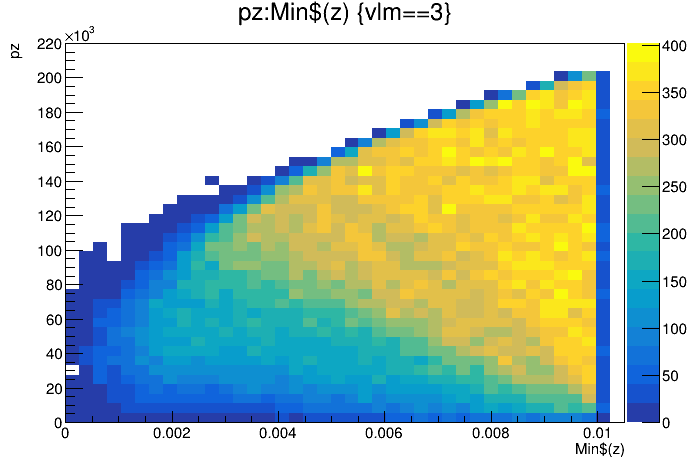# Graphing the average of multiple rows

Hello!
I’m new to root and have recently installed it to work with the data that I’m obtaining from a geant4 particle simulation (Never worked with c++ either so forgive me if I sound like an idiot). My current file structure involves a single tree with 1e+6 rows/entries (1 for each particle). Then for each row there iterations/instances for each “step” of that particle (point where they are created, change direction, , hit an atom, etc). What I want to be able to do is to be able to interpret the average final momentum (through a 2d histogram or a graph) for the starting point of a particle. At the moment I can use the command in the root terminal

t->Draw(“pz:Min\$(z)”,“vlm==3”)

pz is the momentum of a particle (in Z axis) at a given instance/iteration, and vlm = 3 means the particle makes it to the detector. Min\$(z) gives the lowest z value of all the iterations for the row that a pz’s iteration/instance belongs too. So basically, this command graphs for me the final momentum of a particle vs its starting position inside the source.Vertical Axis = particle momentum
Horizontal Axis = position of particle creation (min\$(z)

What I need to do is find the average final (hitting vlm 3) momentum of all the particles for a given min\$(z), not graph the momentum of every single particle against its min\$(z). I tried to use the sum\$ and length\$ commands to find the mean, but these only work for all the iterations in a row, not all the rows (it gives me the average pz of all the steps of a certain particle vs starting position, not the average final step pz of all the particles). I have spent the last few days working on this but cannot find a solution and am really struggling so I can hear. Could someone please give me some help?

Thanks,
Rio

To me, this seems like a task that should be easily accomplished using RDataFrame. Please, check the documentation at Dataframes - ROOT and the tutorials at ROOT: Dataframe tutorials. The full reference guide can be found at https://root.cern/doc/master/classROOT_1_1RDataFrame.

Cheers,
J.